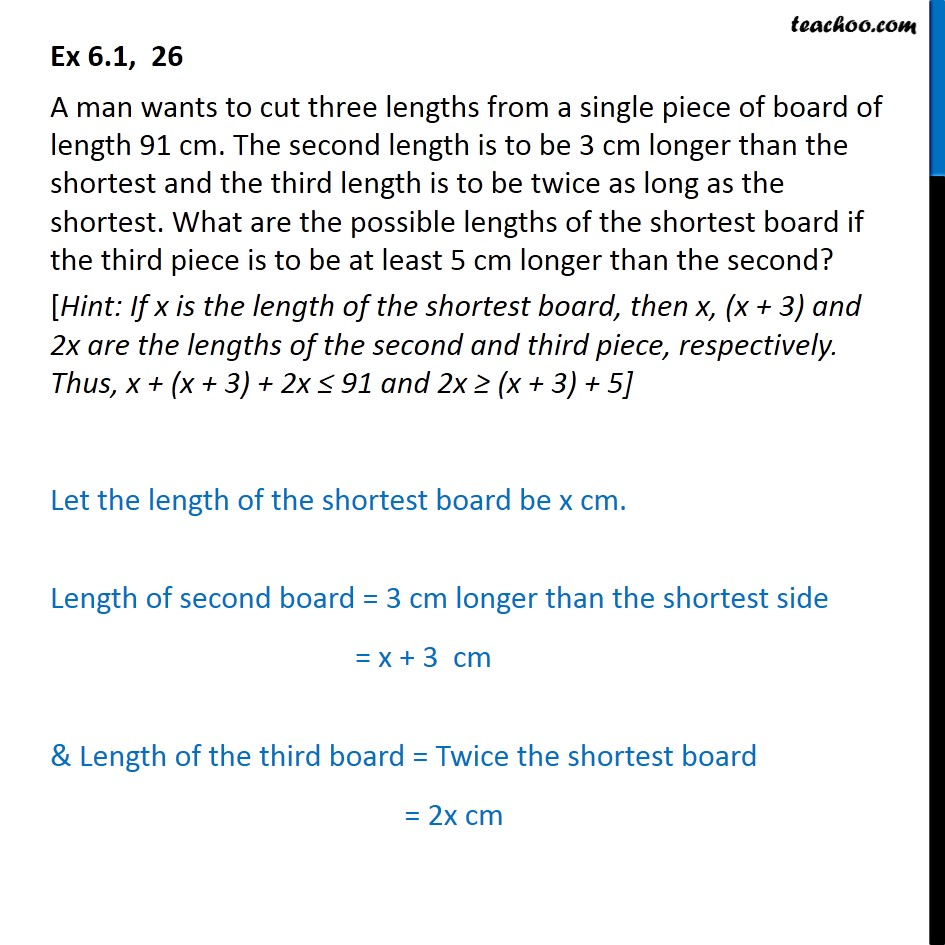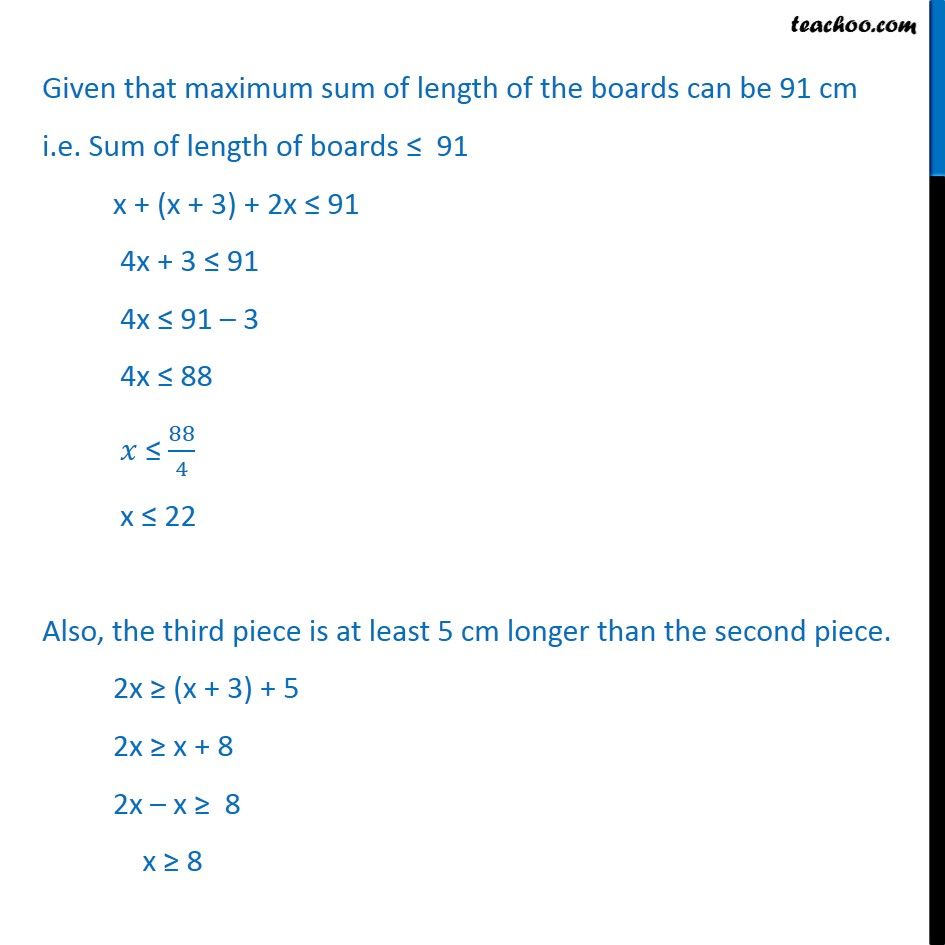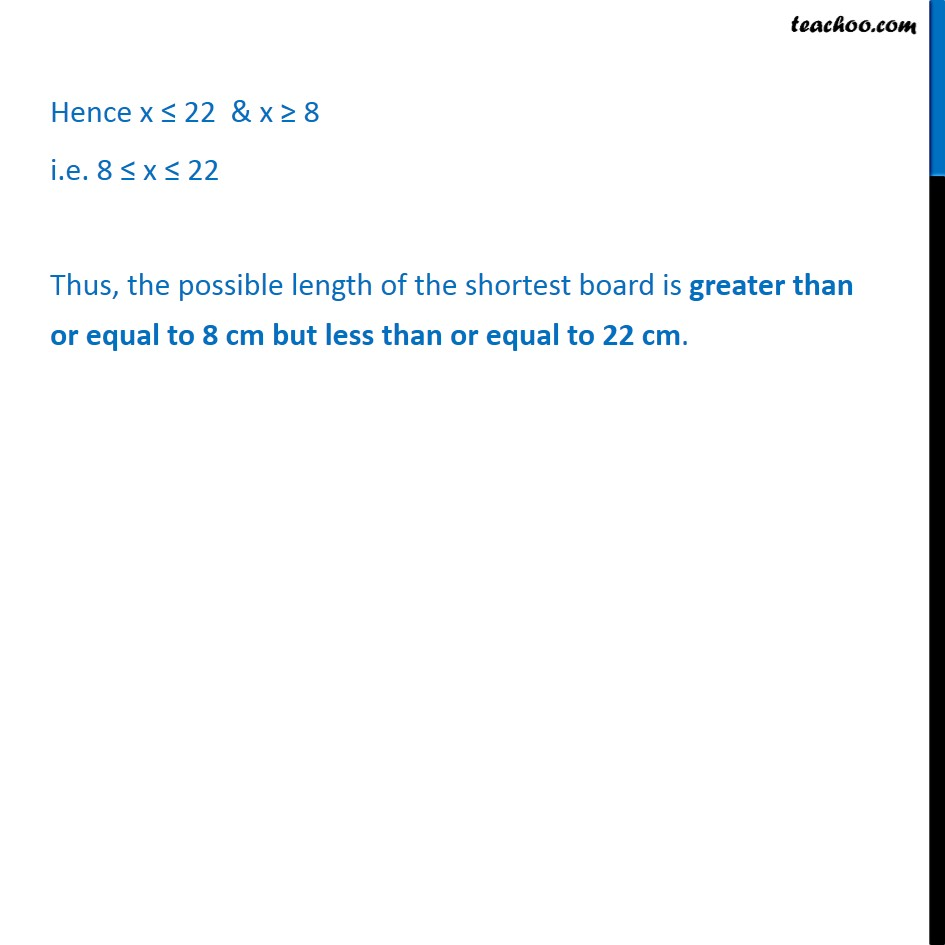Ex 5.1

Chapter 5 Class 11 Linear Inequalities
Serial order wiseLearn in your speed, with individual attention - Teachoo Maths 1-on-1 Class

### Transcript

Ex 5.1, 26 A man wants to cut three lengths from a single piece of board of length 91 cm. The second length is to be 3 cm longer than the shortest and the third length is to be twice as long as the shortest. What are the possible lengths of the shortest board if the third piece is to be at least 5 cm longer than the second? [Hint: If x is the length of the shortest board, then x, (x + 3) and 2x are the lengths of the second and third piece, respectively. Thus, x + (x + 3) + 2x ≤ 91 and 2x ≥ (x + 3) + 5] Let the length of the shortest board be x cm. Length of second board = 3 cm longer than the shortest side = x + 3 cm & Length of the third board = Twice the shortest board = 2x cm Given that maximum sum of length of the boards can be 91 cm i.e. Sum of length of boards ≤ 91 x + (x + 3) + 2x ≤ 91 4x + 3 ≤ 91 4x ≤ 91 ­– 3 4x ≤ 88 𝑥 ≤ ﷐88﷮4﷯ x ≤ 22 Also, the third piece is at least 5 cm longer than the second piece. 2x ≥ (x + 3) + 5 2x ≥ x + 8 2x – x ≥ 8 x ≥ 8 Hence x ≤ 22 & x ≥ 8 i.e. 8 ≤ x ≤ 22 Thus, the possible length of the shortest board is greater than or equal to 8 cm but less than or equal to 22 cm.